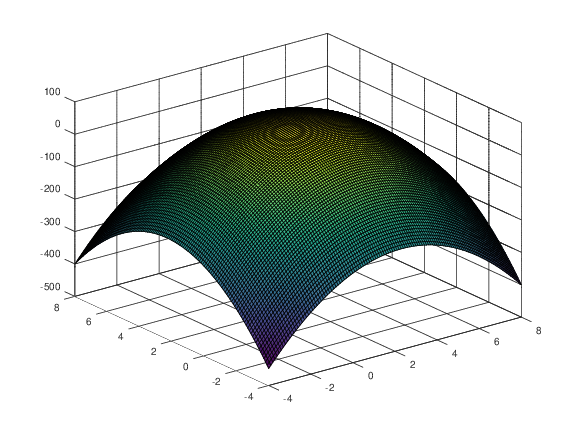UNB/ CS/ David Bremner/ teaching/ cs2613/ labs/ Lab 19

# Before the lab

• Complete any needed linear algebra review

# Working with grids

Time
15 minutes
Activity
Demo
• Octave has as special notation for ranges that we can use to initialize arrays.

``````  r = [-1:0.5:1]
``````
• The builtin function meshgrid can be used to take Cartesian Products of two arrays.

``````  [X,Y] = meshgrid(r,r)
``````
• Despite what best practice is in languages like Java, Octave is quite fond of parallel arrays. In this example, the `(r(i),r(j))` element of the cartesian product is `[X(j,i),Y(j,i)]`. Since we just care about hitting all combinations, we won't worry too much about flipping indices

• For each point in our grid, we can compute a height as follows

``````  Z = X.^2 - Y.^2
``````
• We can then plot a surface using those heights with

``````  surf(X,Y,Z)
``````
• Things are very blocky here because we don't have many points. This is so we can understand the intermediate matrices, and how `surf` works. We'll use more points to give a smoother appearence in what follows.

# Defining and testing a function

Time
20 minutes
Activity
individual

In the rest of this lab we want to use Octave to explore the function

``````    δ(β, a, b) = βa·b - (β-1)b·b
``````

Here `β` is a scalar (a number) and `a` and `b` are vectors of length 2. We curious what the zeros of this function are for fixed `β` and `a`. One approach is to try plotting that surface as a function of `b`. We'll start by defining and testing a function for `beta`

• Translate the mathematical function `δ` into an Octave function.
```    ## compute βa·b - (β-1)b·b
## a, b are assumed to be column vectors, beta is a scalar
function ret = delta(beta, a, b)

endfunction
```
• Complete the tests according to given comments. Use `rand` to generate `a` and `b`. You will also have to look up the documentation for `assert` for how have a floating point error tolerance.
```%% delta(0,a,b) = |b|²
%!test
%!
%!
%!

%% delta(1,a,b) = a.b
%!test
%!
%!
%!

%% delta(2,a,b) = 2a.b - |b|²
%!test
%!
%!
%!
```

# Making 3D plots

Time
20 minutes
Activity
Individuals
• Write one or more for loops to initialize `Z`
```a = [4;4];
beta = 7.5;

%% Generating vectors
range = [-4:0.1:8];

% Compute cartesian product (grid)
[X,Y] = meshgrid(range,range);

surf(X,Y,Z);
```
• The resulting plot should look something like the following.• Try replacing `surf` with `contourf` to get a different view of the surface. You can also "zoom" by changing `range`, but change it back for the timing tests below. What can you guess about the zero set (where the surface intersects the `z=0` plane).

# Python Quiz

• Questions will be distributed on paper at or before 9:30.